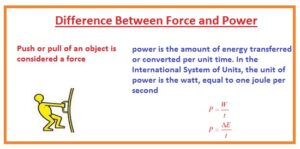Hello guys, I hope you all are doing great. In today’s tutorial, we will discuss the Difference Between Force and Power. The force is an action exerted on the object or interaction among 2 objects. While power is the energy used when action is done on the body. The unit in which force measured is newton and pressure measured in watt.

In today’s post, we will have a detailed look at both force and pressure find their different parameters to discuss their differences. So let’s get started with Difference Between Force and Power.

#### Difference Between Force and Power

Force

• The action among 2 objects due to that body changes its position or retains is called force.
• Force is a term that used to vary the movement of any body.
• It causes the body to rest and move.
• It also called pull or push.
• It is a vector quantity that means has direction and magnitude.
• Its unit is newton and measure denoted with F
• According to newton’s law force applying at the body is equal to the term through which its momentum varies.
• In the case of contact mass acceleration is in direct relation to the force and inverse to mass
• Its dimension is LMT-2
• F=ma
• The main types of force are contact force and non contact force
• Gravitational force is non-contact.

• Force is denoted as F.
• The formula of force is ma.
• Force is a vector quantity so for its description magnitude and direction is required.
• Its name is due to Isaac Newton who first time gave the concept of force.

Power

The rate of doing work is called power.

• The quantity of energy conversion in a unit called power.
• It measured in watt and joul per second.
• It is scalar terms.
• P=w/t
• It also called any type of activity.
• The output power of ant motor is multiple of torque with the angular speed.
• For a moving car the power is multiple of the force applying at the wheels and speed of car
• On a horse, power is equal to 746 watts. Power also measured in ergs, foot-pound, dBm
• The measuring unit of power is a watt.
• The power is denoted with the P.
• Power is a scalar quantity for its description magnitude is needed.
• Its name is due to James Watt who describes the concept of power.That all about difference between force and power if you have any further query ask in comments. Thanks for reading. Have a good day